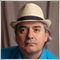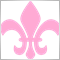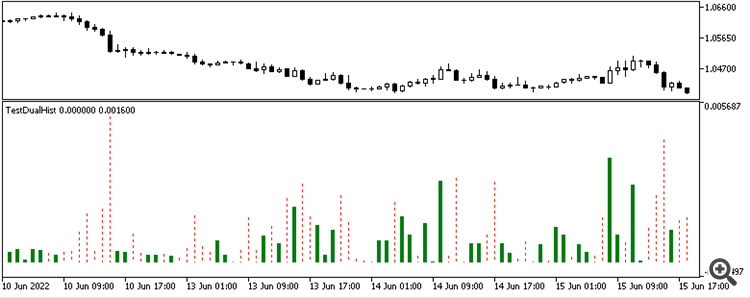# How to overlay Histogram plots in DRAW_HISTOGRAM2 - page 211039

salitos #: Wow then this is a step back, In mql4 this could be achieved just like I was trying, using 2 buffers each having different styles overlaid on each other. Is there any way you may know of differentiating the histogram bars in mql5 apart from using different colors?

I believe you and Vladimir are having miscommunication problems. I am going to attempt to explain both sides.

As he said, you can't mix styles for a single plot, but you can have different styles for separate plots.

So, yes, just as you do on MT4 you can have 2 separate plots, each one being a histogram but with different display properties. Just make sure that they don't both plot values at the same bar index. When one of the plots has value, the other should be EMPTY_VALUE and vice versa. Also, the the plots will share the same scale, so make sure that the values for each plot are comparable.

Also, please remember that in MT4 each buffer corresponded to a single plot, but in MT5 some plot types can be comprised of multiple buffers, usually (but not always) in the form of one buffer for the data and the other for the colour. So make sure that your display properties are indexed based on the plot index and not the buffer index.11039

A few more things:

• Make sure all your "calculation" buffers are registered at the end. Don't mix data and calculation buffers in the sequence.
• For the "Histogram2" type, you need two buffers per plot, not just one. One buffer for the starting position and second buffer for the ending position.
EDIT: However, if you a plotting in a separate window where the Histogram is always zero based, then use the standard "Histogram" with a single buffer. There is no need to use "Histogram2" which allows for a separate start and end values.47

HI Fernando, thanks yes you've really understood the problem and bridged the miscommunication. I have tried setting EMPTY_VALUE rather than zero in the indexes where the first buffer should not plot but this results in my existing(only ADX Buffer)plots completely disappearing. Here is the code I have modified in onCalculate()
```   for(int i=start; i<rates_total; i++)
{

}```

I've attached the empty histogram too

Files:47

Fernando Carreiro #:

A few more things:

• Make sure all your "calculation" buffers are registered at the end. Don't mix data and calculation buffers in the sequence.
• For the "Histogram2" type, you need two buffers per plot, not just one. One buffer for the starting position and second buffer for the ending position.
EDIT: However, if you a plotting in a separate window where the Histogram is always zero based, then use the standard "Histogram" with a single buffer. There is no need to use "Histogram2" which allows for a separate start and end values.
As for using Histogram in place of Histogram2 would the former be enough to support 2 buffers with different styles. If this works, I want the ADX buffer to have different line width from the Awesome Oscillator Buffer.11039

salitos #: HI Fernando, thanks yes you've really understood the problem and bridged the miscommunication. I have tried setting EMPTY_VALUE rather than zero in the indexes where the first buffer should not plot but this results in my existing(only ADX Buffer)plots completely disappearing. Here is the code I have modified in onCalculate()

I've attached the empty histogram too

I suggest you supply the full code instead of just snippets. It is rather difficult for us to pinpoint what you may be doing wrong from just pieces of the code. It's easier when we can see the "full picture" as well as actually run it and see the results.11039

salitos #: As for using Histogram in place of Histogram2 would the former be enough to support 2 buffers with different styles. If this works, I want the ADX buffer to have different line width from the Awesome Oscillator Buffer.

Yes, "Histogram" would be equivalent to the MT4 implementation. "Histogram2" is not for two plots or different styles, but for a single plot where the histograms are NOT zero based.

Supply full code and I will make adjustments so you can see how to do it.47

Sure , I had included snippets because that was the only part I had modified from the last full code I posted on post #3(so as to reduce spam).
```//+------------------------------------------------------------------+
//|                                                  ll.mq5 |
//|                                  Copyright 2022, MetaQuotes Ltd. |
//|                                             https://www.mql5.com |
//+------------------------------------------------------------------+
#property version   "1.00"
#property strict

#property indicator_separate_window
#property indicator_buffers 4
#property indicator_plots   1
//--- plot Histogram_2
#property indicator_label1  "Histo"
#property indicator_type1   DRAW_HISTOGRAM2
#property indicator_color1  clrRed
#property indicator_style1  STYLE_SOLID
#property indicator_width1  1
//+------------------------------------------------------------------+
//| Custom indicator initialization function                         |
//+------------------------------------------------------------------+
double Awesome_Buffer[];
double Awesome_Intermediate_Buffer[];

int bars_calculated=0;
//+------------------------------------------------------------------+
//|                                                                  |
//+------------------------------------------------------------------+
int OnInit()
{
//--- indicator buffers mapping
SetIndexBuffer(2,Awesome_Buffer,INDICATOR_DATA);
SetIndexBuffer(3,Awesome_Intermediate_Buffer,INDICATOR_CALCULATIONS);
//--- Set an empty value

PlotIndexSetDouble(0,PLOT_EMPTY_VALUE,EMPTY_VALUE);
//---
handle=iAO(_Symbol,_Period);
return(INIT_SUCCEEDED);
}
//+------------------------------------------------------------------+
//| Custom indicator iteration function                              |
//+------------------------------------------------------------------+
int OnCalculate(const int rates_total,
const int prev_calculated,
const datetime &time[],
const double &open[],
const double &high[],
const double &low[],
const double &close[],
const long &tick_volume[],
const long &volume[],
{
if(prev_calculated<=0)
{
ArrayInitialize(Awesome_Buffer,0);
ArrayInitialize(Awesome_Intermediate_Buffer,0);
}

handleValuesToBuffer(handle,prev_calculated, rates_total,Awesome_Intermediate_Buffer);

//--- Calculate the indicator values
int start=0;
//--- If already calculated during the previous starts of OnCalculate
if(prev_calculated>0)
start=prev_calculated-1;

for(int i=start; i<rates_total; i++)
{

}

//--- return value of prev_calculated for next call
return(rates_total);

}
//+------------------------------------------------------------------+
int handleValuesToBuffer(const int indi_handle,const int prev_calculated,const int rates_total,double &destBuffer[])
{

//--- number of values copied from the iMA indicator
int values_to_copy;
//--- determine the number of values calculated in the indicator
int calculated=BarsCalculated(indi_handle);
if(calculated<=0)
{
PrintFormat("BarsCalculated() returned %d, error code %d",calculated,GetLastError());
return(0);
}

//--- if it is the first start of calculation of the indicator or if the number of values in the iMA indicator changed
//---or if it is necessary to calculated the indicator for two or more bars (it means something has changed in the price history)
if(prev_calculated==0 || calculated!=bars_calculated || rates_total>prev_calculated+1)
{
//--- if the iMABuffer array is greater than the number of values in the iMA indicator for symbol/period, then we don't copy everything
//--- otherwise, we copy less than the size of indicator buffers
if(calculated>rates_total)
values_to_copy=rates_total;
else
values_to_copy=calculated;
}

else
{
//--- it means that it's not the first time of the indicator calculation, and since the last call of OnCalculate()
//--- for calculation not more than one bar is added
values_to_copy=(rates_total-prev_calculated)+1;
}

CopyBuffer(indi_handle,0,0,values_to_copy,destBuffer);

//--- memorize the number of values in the Moving Average indicator
//   bars_calculated=calculated;

return 0;
}
//+------------------------------------------------------------------+
```11039

salitos #: Sure , I had included snippets because that was the only part I had modified from the last full code I posted on post #3(so as to reduce spam).

Your code is only for one Histogram plot? Where is your attempt at two histogram plots? Do it as if it were MT4 using standard "Histogram".

You also did not correct the points I explained, such as not mixing the "Data" and the "Calculation" buffers. You were warned that ADX and Awesome Oscillator do not share the same scale so they will not plot correctly together.

Please understand, that I am willing to guide and help you, but not to totally recode it for you. So, please, fix the mentioned points first then resupply your new code.47

Fernando Carreiro #:

You also did not correct the points I explained, such as not mixing the "Data" and the "Calculation" buffers. You were warned that ADX and Awesome Oscillator do not share the same scale so they will not plot correctly together.

I think its best If we tackled the issues in isolation as I seem to have failed to grasp something fundamental. Furthermore I had not read your reply #16 at the time of answering. So for the highlighted text I have still not grasped what you mean by mixing the calculation and data buffers, do you mean assign the calculation buffer index value to a scalar before I assign to the Data Buffer index? For the scale, I had tried to address this by assigning the second buffer(Awesome Buffer) to static value "20"(in oncalculate for loop) which should place it somewhere within the scale of the ADX for test purposes, was that sufficient?

I have changed to Histogram as per #16 and the histogram now plots but I cannot see the bars for values of the second buffer(20) for which I have also added a PlotSetInteger for the width property in onInit()

```//+------------------------------------------------------------------+
//|                                                  ll.mq5 |
//|                                  Copyright 2022, MetaQuotes Ltd. |
//|                                             https://www.mql5.com |
//+------------------------------------------------------------------+
#property version   "1.00"
#property strict

#property indicator_separate_window
#property indicator_buffers 4
#property indicator_plots   1
//--- plot Histogram_2
#property indicator_label1  "Histo"
#property indicator_type1   DRAW_HISTOGRAM
#property indicator_color1  clrRed
#property indicator_style1  STYLE_SOLID
#property indicator_width1  1
//+------------------------------------------------------------------+
//| Custom indicator initialization function                         |
//+------------------------------------------------------------------+
double Awesome_Buffer[];
double Awesome_Intermediate_Buffer[];

int bars_calculated=0;
//+------------------------------------------------------------------+
//|                                                                  |
//+------------------------------------------------------------------+
int OnInit()
{
//--- indicator buffers mapping
SetIndexBuffer(2,Awesome_Buffer,INDICATOR_DATA);
SetIndexBuffer(3,Awesome_Intermediate_Buffer,INDICATOR_CALCULATIONS);
//--- Set an empty value

PlotIndexSetDouble(0,PLOT_EMPTY_VALUE,EMPTY_VALUE);
//awesome osc bars should be dotted
PlotIndexSetInteger(2,PLOT_LINE_STYLE,STYLE_DASHDOT);
//---
handle=iAO(_Symbol,_Period);
return(INIT_SUCCEEDED);
}
//+------------------------------------------------------------------+
//| Custom indicator iteration function                              |
//+------------------------------------------------------------------+
int OnCalculate(const int rates_total,
const int prev_calculated,
const datetime &time[],
const double &open[],
const double &high[],
const double &low[],
const double &close[],
const long &tick_volume[],
const long &volume[],
{
if(prev_calculated<=0)
{
ArrayInitialize(Awesome_Buffer,0);
ArrayInitialize(Awesome_Intermediate_Buffer,0);
}

handleValuesToBuffer(handle,prev_calculated, rates_total,Awesome_Intermediate_Buffer);

//--- Calculate the indicator values
int start=0;
//--- If already calculated during the previous starts of OnCalculate
if(prev_calculated>0)
start=prev_calculated-1;

for(int i=start; i<rates_total; i++)
{

//attempt at assigning buffer value to a scalar first to avoid mixing

}

//--- return value of prev_calculated for next call
return(rates_total);

}
//+------------------------------------------------------------------+
int handleValuesToBuffer(const int indi_handle,const int prev_calculated,const int rates_total,double &destBuffer[])
{

//--- number of values copied from the iMA indicator
int values_to_copy;
//--- determine the number of values calculated in the indicator
int calculated=BarsCalculated(indi_handle);
if(calculated<=0)
{
PrintFormat("BarsCalculated() returned %d, error code %d",calculated,GetLastError());
return(0);
}

//--- if it is the first start of calculation of the indicator or if the number of values in the iMA indicator changed
//---or if it is necessary to calculated the indicator for two or more bars (it means something has changed in the price history)
if(prev_calculated==0 || calculated!=bars_calculated || rates_total>prev_calculated+1)
{
//--- if the iMABuffer array is greater than the number of values in the iMA indicator for symbol/period, then we don't copy everything
//--- otherwise, we copy less than the size of indicator buffers
if(calculated>rates_total)
values_to_copy=rates_total;
else
values_to_copy=calculated;
}

else
{
//--- it means that it's not the first time of the indicator calculation, and since the last call of OnCalculate()
//--- for calculation not more than one bar is added
values_to_copy=(rates_total-prev_calculated)+1;
}

CopyBuffer(indi_handle,0,0,values_to_copy,destBuffer);

//--- memorize the number of values in the Moving Average indicator
//   bars_calculated=calculated;

return 0;
}
//+------------------------------------------------------------------+
```
Files:
histopic.PNG  5 kb11039

Here is an example:```//--- Setup

#property indicator_separate_window

// Define number of buffers and plots
#property indicator_buffers   3
#property indicator_plots     2

// Define visual appearance of plots

#property indicator_label1  "Close Up"
#property indicator_type1   DRAW_HISTOGRAM
#property indicator_color1  clrGreen
#property indicator_style1  STYLE_SOLID
#property indicator_width1  5

#property indicator_label2  "Close Down"
#property indicator_type2   DRAW_HISTOGRAM
#property indicator_color2  clrRed
#property indicator_style2  STYLE_DOT
#property indicator_width2  1

//--- Global variable declarations

// Indicator buffers

//--- Event handling functions

// Initialisation event handler
int OnInit(void)
{
return INIT_SUCCEEDED;
};

// Calculation event handler
int
OnCalculate(
const int      rates_total,
const int      prev_calculated,
const datetime &time[],
const double   &open[],
const double   &high[],
const double   &low[],
const double   &close[],
const long     &tick_volume[],
const long     &volume[],
)
{
// Main loop — fill in the arrays with data values
for( int i = prev_calculated < 1 ? 0 : prev_calculated - 1;
i < rates_total; i++ )
{
double dbCloseDelta = close[ i ] - open[ i ];
g_adbCloseUp[    i ] = dbCloseDelta > 0 ?  dbCloseDelta : EMPTY_VALUE;
g_adbCloseDown[  i ] = dbCloseDelta < 0 ? -dbCloseDelta : EMPTY_VALUE;